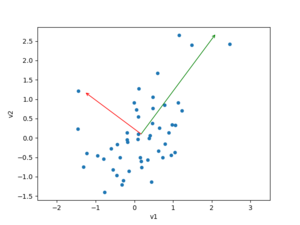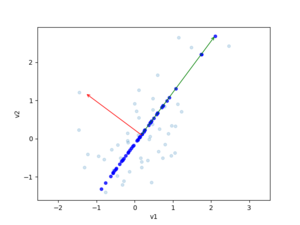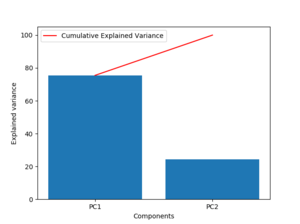# Course:CPSC522/Principal Component Analysis

Jump to navigation Jump to search

## Principal Component Analysis

Principal Component Analysis (PCA) is a dimensionality-reduction technique that is primarily used to represent high-dimensional data sets in fewer dimensions while preserving as much information as possible.

Principal Author: Alireza Iranpour

## Abstract

High-dimensional data sets are increasingly common in a variety of disciplines and are often difficult to interpret. Principal Component Analysis (PCA) is a mathematical procedure for transforming such data sets into a lower-dimensional subspace that captures as much of the variance in the data as possible. In essence, PCA provides a low-dimensional summary of the data.

### Builds on

Dimensionality reduction and feature extraction.

## Content

### Motivation 

Dealing with large data sets with a large number of variables is often challenging. In order to handle the curse of dimensionality and mitigate the risk of overfitting, dimensionality-reduction methods like PCA are widely used. PCA reduces the dimension of the data by combining correlated variables together to form a smaller set of artificial variables called “principal components” that explain most of the variance in the data. This simplification facilitates description, visualization and perception.

PCA is also used as preprocessing prior to running machine-learning algorithms on the data. Most classification and regression models will run significantly faster if they have fewer variables to look at. Moreover, reducing the dimensionality of the data set reduces the number of degrees of freedom, which lowers the risk of overfitting to the data.

### Overview

PCA is an unsupervised learning method that discovers hidden patterns and structures in the data without reference to any prior knowledge. PCA reduces the number of dimensions by geometrically projecting the data onto abstract dimensions called principal components (PCs). The goal here is to find a limited number of principal components that best describe the data. PCs are determined in order of retaining the variation present in the original data. The first PC is chosen to account for the maximum variation. This is done by minimizing the total distance between the original data and their projections which also results in maximum variance in the projected points. The second and subsequent PCs are chosen in a similar way to account for the remaining variance unexplained by the previous PCs. Each PC must be independent of all the previous ones. This independence or lack of correlation is referred to as orthogonality. If a PC is not orthogonal (perpendicular) to all the previous PCs, then either the current PC does not account for as much variance as possible, or the previous ones failed to do so.

### How PCA works 

Before diving into the details of the algorithm, let’s get a general overview of what PCA does:

First, we calculate a matrix that describes how all of the original variables (features) relate to one another in the high-dimensional space. This matrix is then broken down into two separate components, namely directions and magnitudes. Directions are basically combinations of the original variables, and the magnitudes indicate the variance that each direction can explain, thus showing their importance. Based on the magnitudes, we can identify the most important directions and project our data into a smaller space by excluding those directions that are of least importance.

Now that we have a general idea of PCA, we can go over the details:

In order to conduct a principal component analysis, we need to have a high-dimensional data set with $n$rows and $d+1$columns where one column corresponds to the dependent variable or target $(Y)$while the other $d$columns each correspond to an independent variable or feature $(X)$.

1. As mentioned before, PCA is an unsupervised learning method that does not take into account the dependent variable. In this regard, the data set needs to be divided into $X$and $Y$. All that PCA cares about is the feature set $X$. If the dependent variable is part of the data set, it needs to be separated.

$Dataset\ \rightarrow \ X\ and\ Y$2. We center our data simply by subtracting the mean of each column (feature) from each of the observations in that column. This converts the mean of our data into zero.

3. We could also standardize the features by dividing each observation by the standard deviation of its column.

Steps 2 and 3 transform the matrix of features $(X)$into another matrix called $Z$. Matrix $Z$is the standardized version of the matrix $X$.Eigenvectors that maximize the variance explained. The first vector (in green) captures most of the variance. The remaining variance is then accounted for by the second vector (in red), which is orthogonal to the first one.

4. Using matrix $Z$, we can calculate the previously mentioned matrix of variable relationships called the covariance matrix as follows:

$Covariance\ Matrix=Z^{T}Z$5. Based on the resulting covariance matrix, we will compute the eigenvectors and their corresponding eigenvalues. This is referred to as eigendecomposition of the matrix $Z^{T}Z$where we decompose $Z^{T}Z$into $Q\Lambda Q^{T}$. $Q$is an orthogonal matrix of the eigenvectors, and $\Lambda =diag\ (\lambda _{1},\lambda _{2},...,\lambda _{d})$is a diagonal matrix of the eigenvalues where each eigenvalue on the diagonal corresponds to an eigenvector in $Q$.

We can perform this decomposition in this fashion because $Z^{T}Z$is a symmetric, positive semi-definite matrix.

6. The eigenvalues $(\lambda _{1},\lambda _{2},...,\lambda _{d})$from the diagonal matrix $\Lambda$indicate the magnitude (importance) of each eigenvector (direction). In this regard, we will sort the eigenvectors (columns of $Q$) according to their corresponding magnitude from largest to smallest. We will call this new sorted matrix of eigenvectors $Q^{*}$.Reducing the original feature space into a single dimension by projecting the data onto the axis of the first (most important) PC (the green vector)

7. Finally, we will project our data onto the eigenvectors by calculating $Z^{*}=ZQ^{*}$. The new matrix $Z^{*}$represents our data in terms of the ordered eigenvectors in matrix $Q^{*}$. Since the columns of $Q^{*}$were orthogonal, the columns of $Z^{*}$(PCs) will also be orthogonal. We can choose to keep as many of the columns of this matrix (PCs) as we want and drop the rest.

### Choosing the number of Principal Components

The dimensionality of the subspace into which we project our data can be determined in two different ways:

One way is to choose the dimensions arbitrarily. For instance, if our goal is to visualize the data, we can reduce it into 2 dimensions using only the first two principal components.

Another way is to calculate the proportion of variance explained. We can determine a threshold and keep including more PCs until we reach or exceed the target threshold. The proportion of variance explained by each individual PC can be calculated as:

$Proportion\ of\ Variance\ Explained\ by\ PC_{j}={\frac {\lambda _{j}}{\textstyle \sum _{j=1}^{d}\lambda _{j}\displaystyle }}$Once, we calculate this proportion for each Principal Component, we can sort them accordingly and plot the cumulative proportion of the variance explained as a function of the number of components. This plot helps us determine the number of PCs to include before reaching the target threshold.The first principal component (PC1), which corresponds to the green vector from the previous plot, retains about 75% of the total variance. Clearly, including both PCs would retain 100% of the variance.

### Applications of PCA 

#### Data Compression

By reducing the dimensionality, PCA can compress the data.

#### Outlier Detection

PCA gives poor approximation of samples that are very different from typical observations. In this regard, samples with the highest reconstruction error can be identified as outliers. Reconstruction error is the error made by projecting the data into the new lower-dimensional space. For instance, principal components that have been extracted to describe images of numbers do a terrible job at approximating an image of a cat and can, therefore, identify it as an outlier.

#### Data Visualization

PCA can help visualize high-dimensional data by reducing them into a visualizable subspace. However, for the sole purpose of visualization, alternative algorithms such as MDS or t-SNE are preferred.

#### Data Interpretation

By assigning meaning to the extracted features (PCs), we can make our high-dimensional data more interpretable. For instance, numerous personal characteristics can be abstracted into 5 major traits, also known as OCEAN (Openness, Conscientiousness, Extraversion, Agreeableness, Neuroticism). We can think of each trait as a principal component.

### Weaknesses 

• PCA only allows linear projections. As a result, it might miss non-linear patterns in the data.
• The covariance matrix is a $d\times d$matrix where $d$is the original dimensionality of the data. In this regard, for large values of $d$, calculation of this matrix becomes intractable. This limitation, however, can be resolved by using singular value decomposition (SVD).
• Forces orthogonality to minimize the reconstruction error. Independent component analysis (ICA) is an alternative method that uses information theory to find statistically independent directions.
• Assumes multivariate normal distribution in the data. This limitation can be resolved by using kernel tricks (kernel PCA).

## To Add

Kernel PCA

Singular value decompositionPermission is granted to copy, distribute and/or modify this document according to the terms in Creative Commons License, Attribution-NonCommercial-ShareAlike 3.0. The full text of this license may be found here: CC by-nc-sa 3.0